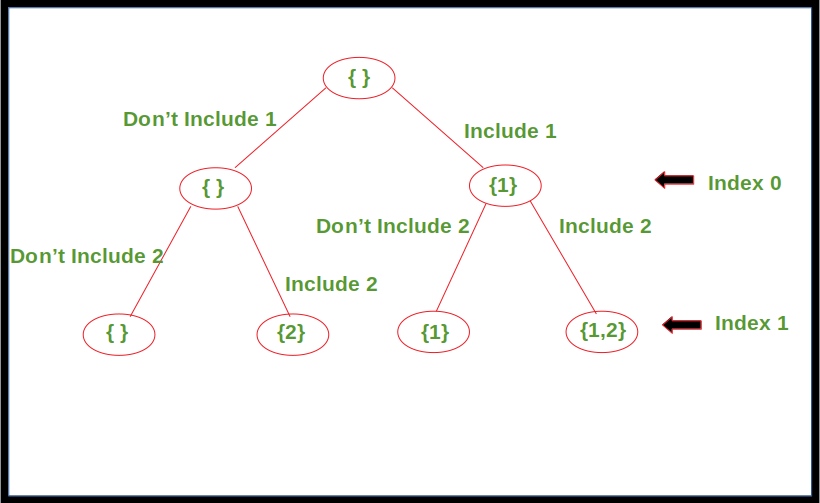Related Articles

# Generating all possible Subsequences using Recursion

• Difficulty Level : Easy
• Last Updated : 21 Oct, 2021

Given an array. The task is to generate and print all of the possible subsequences of the given array using recursion.

Examples:

```Input : [1, 2, 3]
Output : , , [2, 3], , [1, 3], [1, 2], [1, 2, 3]

Input : [1, 2]
Output : , , [1, 2]
```

Approach: For every element in the array, there are two choices, either to include it in the subsequence or not include it. Apply this for every element in the array starting from index 0 until we reach the last index. Print the subsequence once the last index is reached.

Below diagram shows the recursion tree for array, arr[] = {1, 2}.Below is the implementation of the above approach.

## C++

 `// C++ code to print all possible``// subsequences for given array using``// recursion``#include ``using` `namespace` `std;` `void` `printArray(vector<``int``> arr, ``int` `n)``{``    ``for` `(``int` `i = 0; i < n; i++)``        ``cout << arr[i] << ``" "``;``    ``cout << endl;``}` `// Recursive function to print all``// possible subsequences for given array``void` `printSubsequences(vector<``int``> arr, ``int` `index,``                       ``vector<``int``> subarr)``{``    ``// Print the subsequence when reach``    ``// the leaf of recursion tree``    ``if` `(index == arr.size())``    ``{``        ``int` `l = subarr.size();` `        ``// Condition to avoid printing``        ``// empty subsequence``        ``if` `(l != 0)``            ``printArray(subarr, l);``    ``}``    ``else``    ``{``        ``// Subsequence without including``        ``// the element at current index``        ``printSubsequences(arr, index + 1, subarr);` `        ``subarr.push_back(arr[index]);` `        ``// Subsequence including the element``        ``// at current index``        ``printSubsequences(arr, index + 1, subarr);``    ``}``    ``return``;``}` `// Driver Code``int` `main()``{``    ``vector<``int``> arr{1, 2, 3};``    ``vector<``int``> b;` `    ``printSubsequences(arr, 0, b);` `    ``return` `0;``}` `// This code is contributed by``// sanjeev2552`

## Java

 `// Java code to print all possible``// subsequences for given array using``// recursion``import` `java.io.*;``import` `java.util.*;` `class` `GFG{``      ` `// Recursive function to print all``// possible subsequences for given array``public` `static` `void` `printSubsequences(``int``[] arr, ``int` `index,``                                     ``ArrayList path)``{``    ` `    ``// Print the subsequence when reach``    ``// the leaf of recursion tree``    ``if` `(index == arr.length)``    ``{``        ` `        ``// Condition to avoid printing``        ``// empty subsequence``        ``if` `(path.size() > ``0``)``            ``System.out.println(path);``    ``}``    ` `    ``else``    ``{``        ` `        ``// Subsequence without including``        ``// the element at current index``        ``printSubsequences(arr, index + ``1``, path);``        ` `        ``path.add(arr[index]);``        ` `        ``// Subsequence including the element``        ``// at current index``        ``printSubsequences(arr, index + ``1``, path);``        ` `        ``// Backtrack to remove the recently``        ``// inserted element``        ``path.remove(path.size() - ``1``);``    ``}``    ``return``;``}` `// Driver code``public` `static` `void` `main(String[] args)``{``    ``int``[] arr = { ``1``, ``2``, ``3` `};``      ` `      ``// Auxiliary space to store each path``      ``ArrayList path = ``new` `ArrayList<>();``      ` `      ``printSubsequences(arr, ``0``, path);``}``}` `// This code is contributed by Mukul Sharma`

## Python3

 `# Python3 code to print all possible ``# subsequences for given array using ``# recursion``  ` `# Recursive function to print all``# possible subsequences for given array``def` `printSubsequences(arr, index, subarr):``      ` `    ``# Print the subsequence when reach ``    ``# the leaf of recursion tree``    ``if` `index ``=``=` `len``(arr):``          ` `        ``# Condition to avoid printing``        ``# empty subsequence``        ``if` `len``(subarr) !``=` `0``:``            ``print``(subarr)``      ` `    ``else``:``        ``# Subsequence without including ``        ``# the element at current index``        ``printSubsequences(arr, index ``+` `1``, subarr)``          ` `        ``# Subsequence including the element``        ``# at current index``        ``printSubsequences(arr, index ``+` `1``, ``                            ``subarr``+``[arr[index]])``      ` `    ``return``          ` `arr ``=` `[``1``, ``2``, ``3``]``  ` `printSubsequences(arr, ``0``, [])` `#This code is contributed by Mayank Tyagi`

## Javascript

 ``
Output:
```

[2, 3]

[1, 3]
[1, 2]
[1, 2, 3]```

Time Complexity:Attention reader! Don’t stop learning now. Get hold of all the important DSA concepts with the DSA Self Paced Course at a student-friendly price and become industry ready.  To complete your preparation from learning a language to DS Algo and many more,  please refer Complete Interview Preparation Course.

In case you wish to attend live classes with experts, please refer DSA Live Classes for Working Professionals and Competitive Programming Live for Students.

My Personal Notes arrow_drop_up